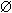Basic notions. Examples of sets

Set. Element of a set. Finite set. Empty set.

Infinite set. Countable set. Uncountable set.

Convex set. Methods of description of sets.

A set and an element of a set concern with category of primary notions , for which it's impossible to formulate the strict definitions. So, we imply as sets usually collections of objects ( elements of a set ), having certain common properties. For instance, a set of books in a library, a set of cars on a parking lot, a set of stars in the sky, a world of plants, a world of animals – these are examples of sets.

A finite set consists of finite number of elements, for example, a set of pages in a book, a set of pupils in a school etc.

An empty set ( its designation is) doesn't contain any elements, for instance, the set of winged elephants, the set of roots of the equation  sin x = 2 etc.

An infinite set consists of infinite number of elements, i.e. this is a set, which isn't finite and empty. Examples: the set of real numbers, a set of points on a plane, a set of atoms in the universe etc.

A countable set is a set, elements of which can be numbered. For example, the sets of natural, even, odd numbers. A countable set can be finite ( a set of books in a library ) or infinite ( the set of integers, its elements can be numbered as follows:

the set elements: …, –5,   – 4,   –3,   –2,   –1,    0,    1,    2,    3,    4,    5, …

their numbers: …   11       9      7      5      3     1     2     4     6     8    10 … ) .

An uncountable set is a set, elements of which can't be numbered. For example, the set of real numbers. An uncountable set can be only infinite ( think, please, why ? ).

A convex set is a set, which for any two its points A and B contains also the whole segment AB . Examples of convex sets: a straight line, a plane, a circle. But a circumference is not a convex set.

Methods of description of sets . A set can be described the following ways:

– an enumeration of all its elements by theirs names ( for example, a set of books in a library, a set of pupils in a class, an alphabet of any language and so on );

– by giving of common performance (common properties) of elements of the set ( for instance, the set of rational numbers, the family of dogs, the family of cats etc.);

– formal law of forming elements of the set ( for example, the formula of a general term of numerical sequence, Periodic table of chemical elements ).